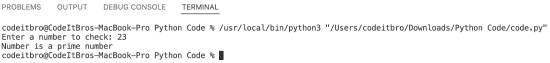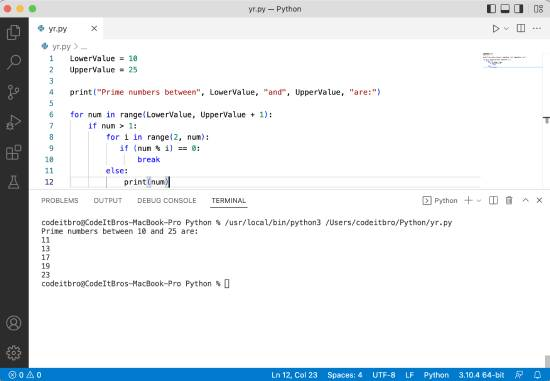# Python Program To Check Prime Number

In this Python program, we will see how to check if a number is prime or not. A prime number is only divisible by one and the number itself. If a given number is divisible by any other number, it is not prime.

By using this logic, we can write a Python program to check if it is prime or not quickly. Before moving ahead, please ensure that you understand Python programming concepts such as variables, conditional statements, for loop, etc.

Read these Python books and enroll in any of these courses to kickstart your career as a Python developer.

## Python Program To Check Prime Number

To check the prime number, you first have to set up a boolean variable (Check) which will keep the status of whether the number is prime or not after checking it with if and for loops.

Here’s the Python code to check whether a number is prime or not.

```# Program to check if a number is prime or not

#Take input from user to check
num = int(input("Enter a number to check: "))

#Setting up a flag to update prime number status
check = False

#Checking if a number is prime or not with conditional and loop statements
if num>1:
for i in range(2, num):
if(num%i) == 0:
check = True
break

#Displaying if a number is prime or not
if check:
print("Number is not a prime number")

else:
print("Number is a prime number")```

Also, check these Python programs:

## Output

After running the code in VS Code, you will get an output similar to this.## Python program to find prime numbers in an interval

• Initialize LowerValue and UpperValue variables to define the interval.
• We will use the for loop to iterate through each number in the interval to check if its prime number or not.
• If the number is prime in the interval, we will display it using the print statement.

Code:

```LowerValue = 10
UpperValue = 25

print("Prime numbers between", LowerValue, "and", UpperValue, "are:")

for num in range(LowerValue, UpperValue + 1):
if num > 1:
for i in range(2, num):
if (num % i) == 0:
break
else:
print(num)```

Output:Also, check these Python programs:

Scroll to Top